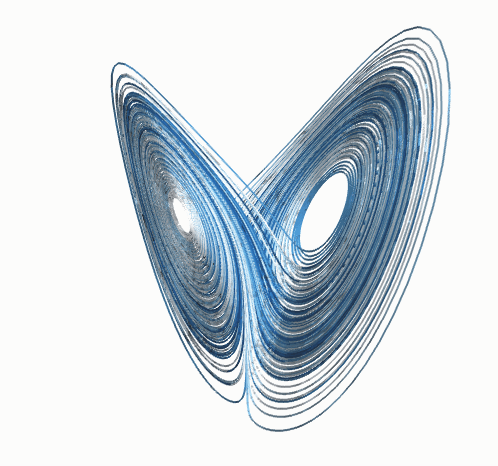# Fun Science: Chaos

The common concept of chaos as the disordered unknown is a concept thousands of years old. Chaos as a branch of mathematics refers to an extremely ordered but complex behavior. In some lights, mathematical chaos might seem utterly random; different inspections reveal order. The first model of chaos emerged in the 1950s to model the movement of air in the atmosphere– the Lorenz model.

### Defining Chaos in Mathematics

First, let’s discuss what chaos is in a mathematical sense (chaos on scholarpedia). In daily language, we would assume that something chaotic is not at all predictable. Chaos is deterministic, which means that if we know the position or value of a chaotic element exactly, then we know every value that it will ever have for all times. Many non chaotic systems are deterministic also; if we measure the position and velocity of a pendulum and its frictional loss, we can predict its path for all time.

If we are measuring some value, there is a limit to our possible accuracy. If you measure your weight, you are measuring your weight plus or minus some error associated with the instrument. We know some scales are more accurate, and some are less accurate. When we measure the position and velocity of the pendulum, there is some error, but the behavior at position x plus a little bit (or x+ε) is essentially identical to the behavior at position x. In a chaotic system, the paths following positions x+ε and x will be entirely different after some window of time. The paths will be similar for some window of time; the length of this window is determined by how chaotic the system is. This behavior is known as sensitive dependence upon initial conditions (or more famously, the butterfly effect).

The lower half of the image below shows the difference between two paths starting at x+ε and x in the Lorenz attractor. The paths are nearly the same, until they quickly become very different. You can look at the Lorenz attractor in more detail and play with it here. The link goes to a Java applet hosted by Caltech where you can change the parameters and see what happens in a time series and in a different representation called the state space.The above image also shows the third of the three most common criteria for chaos: periodicity. This means that the system oscillates in some kind of way. The top half the picture above shows the time series of z variable (see link above for the full model if you are interested). If we plot the data in a different way, we get the image below. This image is called the Lorenz attractor. We see it loops around, but that it is bounded in space. That is, there are places we can say the path will not go. Intriguingly, the Lorenz attractor has a fractal dimension of 2.06. For more on fractals, you can read my posts on fractals and fractal dimensions.### Simple example of chaos: the double pendulum

Many pretty simple systems can exhibit chaos. The easiest example is the double pendulum, which is just a pendulum whose bottom is the top for a second pendulum.  The video below shows the complexity of behavior achieved by the double pendulum. Chaos has also been demonstrated in electrical circuits, biological systems, electrochemical reactions and planetary orbits. In another post, I will write about the amazingly universality of chaos, which shows how all of the diverse systems I listed are in fact very similar.